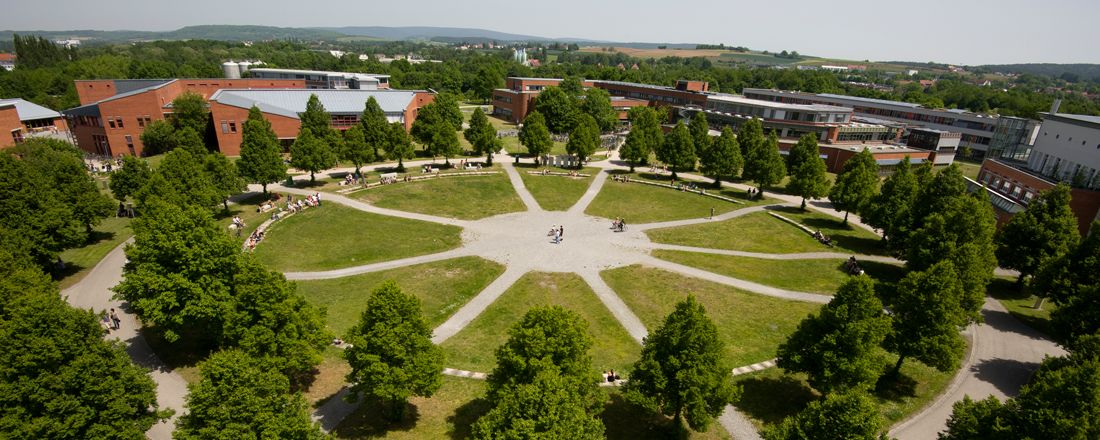## Faculty of Mathematics, Physics & Computer Science

### Chair of Scientific Computing

Print page# Why Scientific Computing?

Over the past several years, numerical simulations of phenomena in technology and the natural sciences have been shown to be an essential tool for accelerating development cycles in industry and businesses. Whereas researchers once had to meticulously study the properties of a product on the basis of prototypes, they are now simulated and optimized on computers. Demands on the capabilities of numerical simulation continue to grow with the need for models that are more and more precise, the incorporation of new problem areas such as data analysis (e.g. big data), and parameter-dependent problems and models with uncertain data. This was triggered by the relatively young and forward-looking research area scientific computing.

## Knowledge transfer

The field addresses the entire solution chain, including modelling; mathematical, numerical, and statistical analysis; optimization; the implementation of algorithms on high-performance computers; and the visualization of results. However, little attention has been paid to training students in this development. Graduates of mathematics are generally still limited to a basic understanding of numerics and scientific computing. Due to the high demands of studying mathematics, there is usually not much time left over to transfer newly acquired knowledge to neighbouring fields that represent intellectual challenges in their own right.

## Fundamental concept

The objective of the international master’s programme is thus to provide a specialized range of courses that leads highly qualified, hard-working students towards the development and mathematical analysis of highly efficient numerical methods. It is a crucial point that highly complex problems are brought to a less complex numerical approximation (on parallel computers) via an understanding of their mathematical core. The master’s programme involves – and is motivated by – a number of courses in other subject areas (biochemistry, physics, computer science, and engineering), in which the simulation of demanding problems plays a crucial role. The programme is geared towards students working at the intersection of mathematics, computer science, and physics. This interdisciplinary approach enables students to achieve and apply their specialized understanding of efficient methods for solving differential and integral equations and analysing large sets of data, and to extend this know-how to other subject areas.

## Preparing for the elite master’s programme Scientific Computing

The master’s programme is mainly concerned with the efficient numerical solution of partial differential equations. Successful applicants are therefore expected to have acquired profound knowledge in the direction of numerical methods for differential equations during their preceding bachelor’s programme, i.e., we strongly recommend attending lectures such as Introduction to Ordinary Differential Equations, Introduction to Higher Analysis, Introduction to Numerical Mathematics, Higher Skills in Numerical Mathematics and if possible Numerical Methods for Differential Equations.

Webmaster: Maximilian Bauer